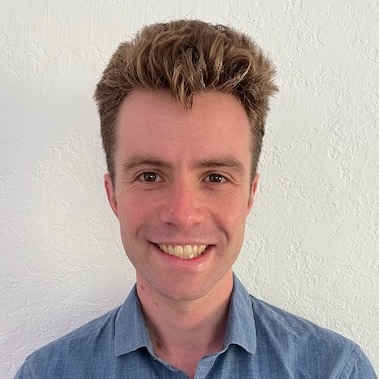Timezone: »

Poster
Quantum Entropy Scoring for Fast Robust Mean Estimation and Improved Outlier Detection
Yihe Dong · Samuel Hopkins · Jerry Li

Tue Dec 10 10:45 AM -- 12:45 PM (PST) @ East Exhibition Hall B + C #228
We study two problems in high-dimensional robust statistics: \emph{robust mean estimation} and \emph{outlier detection}. In robust mean estimation the goal is to estimate the mean $\mu$ of a distribution on $\mathbb{R}^d$ given $n$ independent samples, an $\epsilon$-fraction of which have been corrupted by a malicious adversary. In outlier detection the goal is to assign an \emph{outlier score} to each element of a data set such that elements more likely to be outliers are assigned higher scores. Our algorithms for both problems are based on a new outlier scoring method we call QUE-scoring based on \emph{quantum entropy regularization}. For robust mean estimation, this yields the first algorithm with optimal error rates and nearly-linear running time $\tilde{O}(nd)$ in all parameters, improving on the previous fastest running time $\tilde{O}(\min(nd/\e^6, nd^2))$. For outlier detection, we evaluate the performance of QUE-scoring via extensive experiments on synthetic and real data, and demonstrate that it often performs better than previously proposed algorithms.

#### Author Information

##### Yihe Dong (Microsoft)

Machine learning researcher and engineer interested in geometric deep learning, graph representation learning, and natural language processing.

##### Samuel Hopkins (UC Berkeley)*algorithms, theory of machine learning, semidefinite programming, sum of squares method, bicycles. he/him.* I am a mathematician and computer scientist. I am employed as an Assistant Professor at MIT, in the [Theory of Computing](https://toc.csail.mit.edu/) group in the [Department of Electrical Engineering and Computer Science](https://www.eecs.mit.edu/). Previously, I was a [Miller](http://miller.berkeley.edu/) fellow in the [theory group](http://theory.cs.berkeley.edu/) at UC Berkeley, hosted by [Prasad Raghavendra](https://people.eecs.berkeley.edu/~prasad/) and [Luca Trevisan](https://lucatrevisan.github.io/). Before that, I got my PhD at [Cornell](https://www.cs.cornell.edu/research/theory), advised by [David Steurer](http://www.dsteurer.org/).